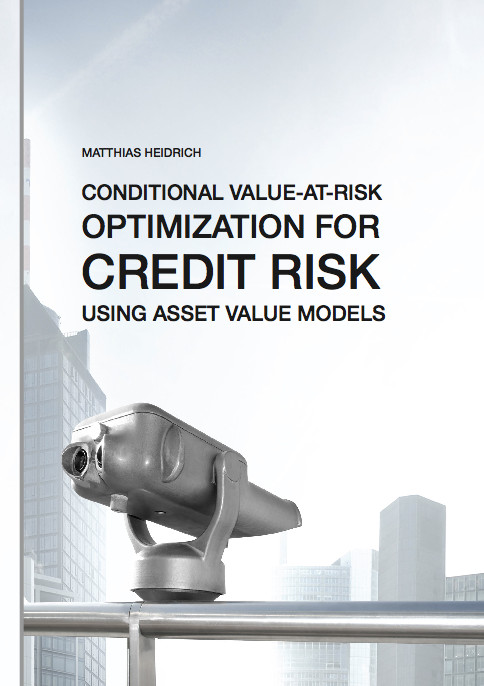Datenbestand vom 15. Mai 2022aktualisiert am 15. Mai 2022

# ISBN 978384390330196,00 € inkl. MwSt, zzgl. Versand

Matthias Heidrich
Conditional Value-at-Risk Optimization for Credit Risk Using Asset Value Models

147 Seiten, Dissertation Technische Universität Darmstadt (2011), Hardcover, B5

## Zusammenfassung / Abstract

Selecting loans from a financial institute's portfolio in order to apply risk reducing credit derivatives or to setup a securitization basket is a binary decision problem. This thesis presents methods to optimize this decision in terms of risk and return. This problem varies from classical portfolio optimization for market-traded assets. Not only due to the integrality condition but also due to the strong non-normality of the loss distribution, a variance-based approach as by Markowitz (1952) is inappropriate.

We follow the work of Rockafellar and Uryasev (2000}, as we formulate risk by Conditional Value-at-Risk (CVaR). It is a linearizable measure of risk when applied to portfolio loss distributions obtained by Monte Carlo methods. We complement the three linear programs of minimizing CVaR, maximizing expected return, and minimizing CVaR minus expected return as considered by Krokhmal et al. (2002) with a fourth non-linear program which minimizes CVaR over expected return. This is comparable to the optimization of the well-known return on risk-adjusted capital (RORAC).

We extend results on the equivalence of these programs from the continuous solution space, as discussed by Krokhmal et al. (2002), as far as possible to integer programming. Furthermore, we suggest replacing the expected return with a more conservative formulation by techniques of robust optimization. Using a CVaR-minimization problem with return constraint as an example, we compare a linear and a quadratic variant of the robustification leading to a mixed integer linear program (MILP) and a mixed integer second order cone program (MISOCP), respectively. We observe that the results of these two programs are quite similar, while (MISOCP) impresses with a much faster computation.

For the first three linear programs, we provide heuristics to compute feasible starting points rapidly. Furthermore, we introduce a heuristic to compute solutions of the non-linear fourth program by repeatedly solving one of the linear programs.

All optimizations are conducted using a Merton's type portfolio model and the two-step importance sampling proposed by Glasserman and Li (2005) in order to reduce variance in the results caused by the Monte Carlo simulation.